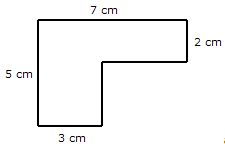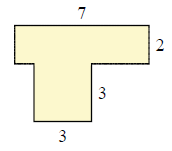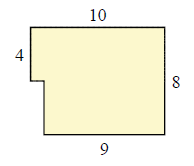# Area of a piecewise rectangular figure

#### Complete Python Prime Pack

9 Courses     2 eBooks

#### Artificial Intelligence & Machine Learning Prime Pack

6 Courses     1 eBooks

#### Java Prime Pack

9 Courses     2 eBooks

In this lesson we find the area of given piecewise rectangular figures. The figures are broken down into two or more rectangles and their areas are found. The sum of the areas of these rectangles gives the area of the piecewise rectangular figure.

Consider the following piecewise rectangular figure. We have to find its area.This figure can be considered as being made of two rectangles of dimensions 7 × 2 and 3 × 3 or 5 × 3 and 4 × 2

Finding the areas of the set of two rectangles gives the area of the piecewise rectangular figure.

Area = 7 × 2 + 3 × 3 14 + 9 = 23 square units

Area = 5 × 3 + 4 × 2 = 15 + 8 = 23 square units

Find the area of piecewise rectangular figure given below.### Solution

Step 1:

Area of a rectangle = l × w; l = length; w = width

Step 2:

Area of 2 piecewise rectangles = 3 × 3 + 7 × 2 = 23 square units

Find the area of piecewise rectangular figure given below.### Solution

Step 1:

Area of a rectangle = l × w; l = length; w = width

Step 2:

Area of 2 piecewise rectangles = 4 × 9 + 10 × 4 = 76 square units